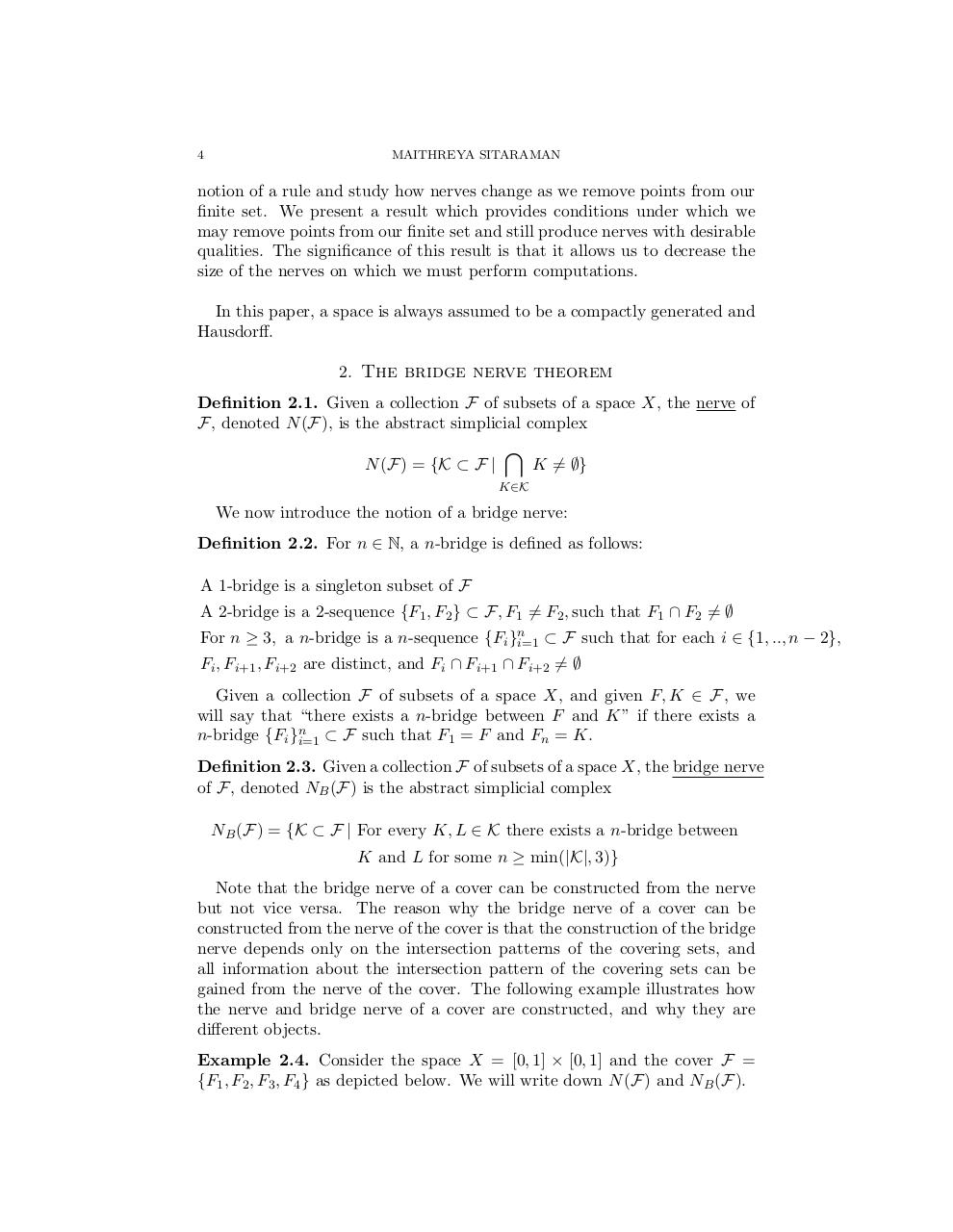# Exploring spaces Oct 10.4.pdfPage 1 2 3 45624

#### Text preview

4

MAITHREYA SITARAMAN

notion of a rule and study how nerves change as we remove points from our
finite set. We present a result which provides conditions under which we
may remove points from our finite set and still produce nerves with desirable
qualities. The significance of this result is that it allows us to decrease the
size of the nerves on which we must perform computations.
In this paper, a space is always assumed to be a compactly generated and
Hausdorff.
2. The bridge nerve theorem
Definition 2.1. Given a collection F of subsets of a space X, the nerve of
F, denoted N (F), is the abstract simplicial complex
N (F) = {K ⊂ F |

\

K 6= ∅}

K∈K

We now introduce the notion of a bridge nerve:
Definition 2.2. For n ∈ N, a n-bridge is defined as follows:
A 1-bridge is a singleton subset of F
A 2-bridge is a 2-sequence {F1 , F2 } ⊂ F, F1 6= F2 , such that F1 ∩ F2 6= ∅
For n ≥ 3, a n-bridge is a n-sequence {Fi }ni=1 ⊂ F such that for each i ∈ {1, .., n − 2},
Fi , Fi+1 , Fi+2 are distinct, and Fi ∩ Fi+1 ∩ Fi+2 6= ∅
Given a collection F of subsets of a space X, and given F, K ∈ F, we
will say that “there exists a n-bridge between F and K” if there exists a
n-bridge {Fi }ni=1 ⊂ F such that F1 = F and Fn = K.
Definition 2.3. Given a collection F of subsets of a space X, the bridge nerve
of F, denoted NB (F) is the abstract simplicial complex
NB (F) = {K ⊂ F | For every K, L ∈ K there exists a n-bridge between
K and L for some n ≥ min(|K|, 3)}
Note that the bridge nerve of a cover can be constructed from the nerve
but not vice versa. The reason why the bridge nerve of a cover can be
constructed from the nerve of the cover is that the construction of the bridge
nerve depends only on the intersection patterns of the covering sets, and
all information about the intersection pattern of the covering sets can be
gained from the nerve of the cover. The following example illustrates how
the nerve and bridge nerve of a cover are constructed, and why they are
different objects.
Example 2.4. Consider the space X = [0, 1] × [0, 1] and the cover F =
{F1 , F2 , F3 , F4 } as depicted below. We will write down N (F) and NB (F).